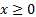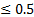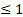/

### Function Description

Returns the cumulative distribution function of the Student’s t distribution. Provided for compatibility with TDIST, T.DIST (with cumulative parameter set to true), T.DIST.2T and T.DIST.RT functions in Microsoft Excel 2010 and later.

Note:

(a)  TDIST is only defined ifand as the Student’s t distribution is symmetric this means that TDIST (1 tailed) isand TDIST (2 tailed) is(b)  T.DIST.RT = 1 - T.DIST(cumulative=true)  and T.DIST(cumulative=true) = MnTDistCdf

(c)   If ifthen TDIST (1 tailed) = T.DIST.RT and TDIST(2 tailed) = 2 * TDIST (1 tailed)

(d)  T.DIST.2T = TDIST (2 tailed)

Please also bear in mind that this function accepts non-integral values of nu whereas the corresponding Excel functions are limited to integral values.

Equivalent to calling MnProbDistCdf using as the distribution parameter “student's t”.

Contents | Prev | Next